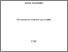Title:

# Vertex diffracted edge waves on a perfectly conducting plane angular sector

Ozturk, Alper Kursat (2009) Vertex diffracted edge waves on a perfectly conducting plane angular sector. PhD thesis, Concordia University.Preview
Text (application/pdf)
NR63422.pdf - Accepted Version
5MB

## Abstract

The problem of electromagnetic scattering from a perfectly-conducting plane angular sector has been of interest for many years. An exact solution for this problem has been developed based on the separation of variables in sphero-conal coordinate system. In this solution, fields and currents are expressed in terms of scalar wave functions that are the solutions of a two parameter eigenvalue problem of two coupled spherical Lame differential equations and spherical Bessel functions. The resulting expressions are in the form of eigenfunction expansions. These expansions are slowly convergent and not suitable for high frequency scattering calculations. Despite their computational advantages, high-frequency modeling techniques fail to provide accurate results for many classes of problems. Lack of a vertex diffraction coefficient is a major factor that limits the accuracy of the high frequency diffraction techniques. Furthermore, the vertex-excited surface currents are strongly guided by the edges of the angular sector creating strong singularities on the current and charge densities. The singular behavior of the current density near the sharp edges of a scattering target is also known as the edge condition and should be modeled properly in numerical solutions to improve the accuracy. In this thesis, numerical diffraction coefficients are derived for vertex-diffracted edge waves induced on an infinitely-thin, perfectly conducting semi-infinite plane angular sector. The diffraction coefficients are formulated to be used in a purely high-frequency modeling of a scattering problem. The current density on the surface of the plane angular sector is modeled using the physical theory of diffraction (PTD). The vertex-diffracted currents are defined as the difference between the exact and PTD currents. The difference current is then modeled as a wave traveling away from the corner with unknown amplitude and phase factors. The unknown coefficients for the vertex-diffracted currents are calculated by using a least squares fit approximation. The vertex-diffracted currents are successfully modeled even for very narrow angular sectors for arbitrary directions of incidence. Illustrative examples are presented to demonstrate the substantial improvement provided by the vertex-diffracted currents to the accuracy of RCS patterns. Another aspect of the research in this thesis is the development of higher-order basis functions for the Method of Moments (MoM) solution. A set of divergence-conforming basis functions was developed to model the singular behavior of the surface currents near the edges and corners of an infinitely-thin, perfectly-conducting polygonal flat plate. The basis functions are derived by imposing the edge and corner conditions on the first order basis functions. It is demonstrated that, even though the new basis functions are not highest order complete, they provide accurate results without incurring complexity in the analysis or additional computational requirements.

Divisions: Concordia University > Gina Cody School of Engineering and Computer Science > Electrical and Computer Engineering Thesis (PhD) Ozturk, Alper Kursat xviii, 124 leaves : ill. ; 29 cm. Concordia University Ph. D. Electrical and Computer Engineering 2009 Paknys, R and Trueman, C. W 976544 Concordia University Library 22 Jan 2013 16:28 18 Jan 2018 17:42 http://clues.concordia.ca/search/c?SEARC...
All items in Spectrum are protected by copyright, with all rights reserved. The use of items is governed by Spectrum's terms of access.

Repository Staff Only: item control pageResearch related to the current document (at the CORE website)
Back to top